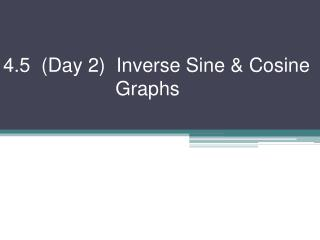Download Presentation4.5 (Day 2) Inverse Sine & Cosine Graphs

# 4.5 (Day 2) Inverse Sine & Cosine Graphs - PowerPoint PPT PresentationDownload Presentation## 4.5 (Day 2) Inverse Sine & Cosine Graphs

- - - - - - - - - - - - - - - - - - - - - - - - - - - E N D - - - - - - - - - - - - - - - - - - - - - - - - - - -
##### Presentation Transcript

1. 4.5 (Day 2) Inverse Sine & Cosine Graphs

2. We learned before about the inverse sine & cosine functions and how their domains need to be restricted. Let’s see why graphically. y = sin x If we simply reflected over y = x we get NOT a function

3. Go back to y = sin x & restrict its domain - want non-repeating values - want positive & negative values Take your paper and trace over the x- & y-axis and the tick marks in Sharpie. Now trace just the part of the curve from Add (+) signs where x-axis & y-axis are positives. Now reflect your paper over y = x. Are (+) in right spots? This is y = Sin–1 x + positive + negative

4. Let’s label! y = Sin–1 x + Check that points are the inverse of points of original sine inversesine + –1 1 YES!! Let’s do the same with cosine, but faster this time! 

5. y = cosx reflect over y = x NOT a function Restrict the domain Want positive & negative & no repeats + positive Take WS & trace axes, tick marks, & section of curve from 0 to π Label positive parts of x- & y-axes + negative

6. Reflect paper (turn it over) Put positives in right places Label your new picture y = Cos–1 x Check that points are the inverse of points of original cosine inversecosine +  (0, 1) (1, 0) (–1, π) YES!! + (–1, π)  (π, –1) –1 1 (1, 0)

7. Now let’s transform Cos–1x and Sin–1x a, b, c, & d still have same effects as before with sin & cos y = a Cos–1b(x – c) + d and y = a Sin–1b(x – c) + d Ex 1) y = Sin–1x + 2 up 2

8. Ex 2) y = 2Sin–1x stretch factor 2 (taller)

9. Ex 3) y = –Cos–1x flip over x-axis

10. Ex 4) y = Cos–1 (½ x) b = ½ “period” wider

11. Homework #406 Pg 220 #20, 21, 32, 34, 35 & Pg 213 Test Yourself (all)